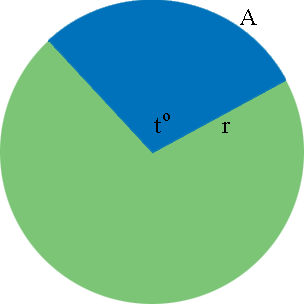SEARCH HOMEMath Central Quandaries & QueriesQuestion from , a student: A reversed curved on a railroad track consists of two circular arcs. The central angle of one is 20 degree with radius 2700 ft and the central angle of the other is 27 degree with radius 3300 ft. Find the total length of the two arcs. thank you po! :)Hi Cherrielyn,

I drew a circle of radius $r$ feet and an arc $A,$ subtended by an angle of $t^o$ at the center of the circle.The circumference of the circle is $2 \pi \; r$ feet. The length of the arc $A$ is a fraction of the circumference and the angle of measure $t^o$ is a fraction of $360^o .$ By the symmetry of the circle you can see that the fractions are the same. For example if $t = 180$ then $t$ is half of $360$ and $A$ is half of the circumference. That is

$A = \frac{180}{360} \times 2 \pi \; r = \frac{1}{2} \times 2 \pi \; r = \pi \; r.$

Likewise if $t = 90$ then

$A = \frac{90}{360} \times 2 \pi \; r = \frac{1}{4} \times 2 \pi \; r = \frac12 \pi \; r.$

The same relationship is true regardless of the value of $t.$ That is in general

$A = \frac{t}{360} \times 2 \pi \; r = \frac{t}{180} \times \pi \; r.$

Your railroad track is made up of two arcs. Find the length of each arc and add them to determine the length of the track.

PennyMath Central is supported by the University of Regina and the Imperial Oil Foundation.